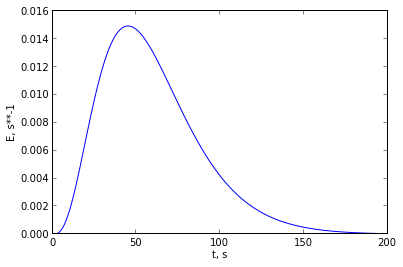Chapter 14 The Tanks-in-Series Model¶

Example 14.1 pageno : 329¶

In :
#Original and new length(m)
import math

# Variables
L1 = 32.            # diameter pipe
L2 = 50.            # pipeline length
sigma1 = 8.         # bottles

# Calculations
# For small deviaqtion from plug flow,sigma_sqr is directly proportional to L
sigma2 = sigma1*math.sqrt(L2/L1);

# Results
print " No of bottles of rose expected is %.f"%(sigma2)
No of bottles of rose expected is 10

Example 14.2 pageno : 330¶

In :
# Variables
sigma1 = 14.        # hours
sigma2 = 10.5;      # hours
L1 = 119.           # ohio miles apart

# Calculations
#spread of curve is directly proportional to sqrt of distance from origin
L = sigma1**2*L1/(sigma1**2-sigma2**2);

# Results
print " The dumping of toxic phenol must have occured within %.f miles upstream of cincinnati"%(L)
The dumping of toxic phenol must have occured within 272 miles upstream of cincinnati

Example 14.3 pageno : 332¶

In :
# Variables
# from figure
vo = 1.;
t1 = 1./6;
t2 = 1.;
t3 = 11./6;
w = 1./10;

# Calculations
A2_by_A1 = 0.5;
R = A2_by_A1/(1-A2_by_A1);
#From the location of 1st peak
V1 = (R+1)*vo*t1;
#From the time between peaks
V2 = (R*vo)*((t2-t1)-(t1));
#From fig 14.3
N = 1+(2*(t1/w))**2;

# Results
print " The reflux ratio is %.f"%(R)
print " The volume of 1st tank is %.3f"%( V1)
print " The volume of 2nd tank is %.3f"%(V2)
print " The number of tanks are %.f "%(N)
The reflux ratio is 1
The volume of 1st tank is 0.333
The volume of 2nd tank is 0.667
The number of tanks are 12

Example 14.4 page no :333¶

In :
%matplotlib inline

from matplotlib.pyplot import *
import math
from numpy import *

# Variables
# from figure E14.4a
t2 = 280.
t1 = 220.
sigma1_sqr = 100.
sigma2_sqr = 1000.

# Calculations
dt = t2-t1;
dsigma_sqr = sigma2_sqr-sigma1_sqr;
N = dt**2/dsigma_sqr;

E = zeros(200)

for t in range(200):
E[t] = ((t**(N-1))*(N**N)*math.exp(-t*N/dt))/((math.factorial(N-1))*(dt**N));

t = zeros(200)
for i in range(200):
t[i] = i;

# Results
plot(t,E)
xlabel("t, s")
ylabel("E, s**-1")
show()
Populating the interactive namespace from numpy and matplotlibIn :